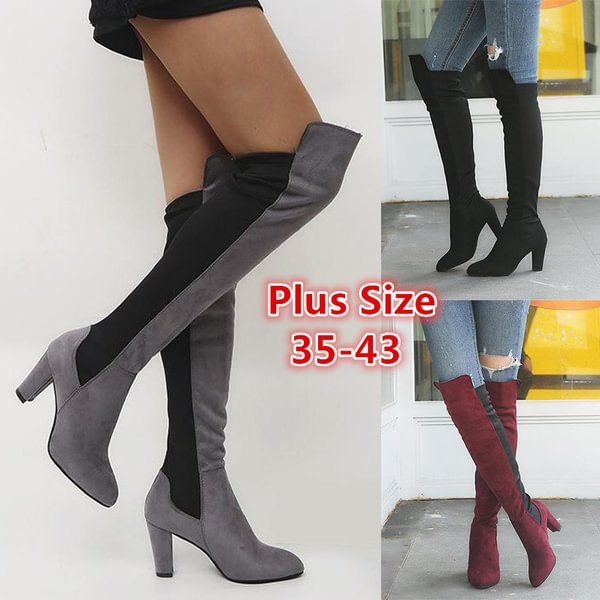Summer Fling 70% UP TO OFF!!!Free Shipping on International Order of \$39+1 / 161 / 16

# Women's Fashion Casual Shoes Long Boots Knee High Boots High Heel Suede High Boots Plus Size 35-43

\$29.00
\$67.00 57% Off
Only left in stock
people are viewing this right now
Get it between -
Color
Size
Other people want this. Over people have this in their bags right now.Size Chart :
4=34=CN(34)=US(4)=EU(34)=220mm(bare feet length)
5=35=CN(35)=US(5)=EU(35)=225mm
5.5=36=CN(36)=US(5.5)=EU(36)=230mm
6=37=CN(37)=US(6)=EU(37)=235mm
7=38=CN(38)=US(7)=EU(38)=240mm
8=39=CN(39)=US(8)=EU(39)=245mm
9=40=CN(40)=US(9)=EU(40)=250mm
10=41=CN(41)=US(10)=EU(41)=255mm
11=42=CN(42)=US(11)=EU(42)=260mm
12=43=CN(43)=US(12)=EU(43)=265mm

Color : Black Grey Red
Material : Suede Rubber

Precautions :
1. Due to manual measurement, please allow an error of 1-3 cm, please refer to the size chart before choosing.
2. Due to the different brightness of the screen, the color may deviate slightly, which is normal.